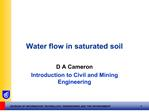DownloadDownload PresentationWater flow in saturated soil

# Water flow in saturated soil

Télécharger la présentation## Water flow in saturated soil

- - - - - - - - - - - - - - - - - - - - - - - - - - - E N D - - - - - - - - - - - - - - - - - - - - - - - - - - -
##### Presentation Transcript

1. Water flow in saturated soil D A Cameron Introduction to Civil and Mining Engineering

3. Head of Water Pressure head = height to which water rises to in a standpipe above the point

4. Confined Aquifer A water bearing layer, overlain and underlain by far less permeable soils.

5. Steady flow in soils � Laminar flow Assumptions to theory: Uniform soil, homogeneous and isotropic Continuous soil media Small seepage flow (non turbulent flow) Darcy�s Law of 1850

6. Darcy�s Law q = kiA � where q = rate of flow (m3/s) i = hydraulic gradient A = area normal to flow direction (m2) k = coefficient of permeability (m/s)

7. Hydraulic Gradient, i

8. Hydraulic Conductivity Coefficient of permeability or just permeability SATURATED soil permeability! Hazen�s formula, for clean and almost uniform sands:

9. TYPICAL PERMEABILITIES Clean gravels > 10-1 m/s Clean sands, sand-gravel 10-4 to 10-2 m/s Fine sands, silts 10-7 to 10-4 m/s Intact clays, clay-silts 10-10 to 10-7 m/s

10. Measuring Permeability [A] Laboratory Constant head test Falling head test Other [B] Field Pumping tests Borehole infiltration tests

11. 1. Constant head permeameter

12. Constant head test Suitable for clean sands and fine gravels EXAMPLE: If the sample area is 4500 mm2, the vertical distance between the 2 standpipe points is 100 mm, ?h is 75 mm Outflow is 1 litre every minute What is the coefficient of permeability?

13. Solution 1000 cm3/min OR q = 16.7 cm3/sec = 16.7x10-6 m3/sec i = 75/100 = 0.75 k = q/(iA) = (16.7x10-6)/(0.75x4500x10-6) m/sec k = 5 x 10-3 m/sec Typically a clean sand or gravel permeability

15. Falling head test Suited to low permeability materials silts and clays Soil sample length, L, and area, A Flow in the tube = flow in the soil

16. 3. Field testing � drawdown test

17. Drawdown test Needs a well-defined water table and confining boundary Must be able to pull down water table and create flow (phreatic line = uppermost flow line)

18. Solution Axi-symmetric problem By integration of Darcy�s Law,

19. TUTORIAL PROBLEMS A canal and a river run parallel, an average of 60 m apart. The elevation of water in the canal is 200 m and the river 193 m. A stratum of sand intersects both the river and canal below the water levels. The sand is 1.5 m thick and is sandwiched between strata of impervious clay. Compute the seepage loss from the canal in m3/s per km length of the canal, given the permeability of the sand is 0.65 mm/s.

21. SOLUTION q = kiA k = 0.65 mm/s = 0.65 x 10-3 m/s ?h = 7 m q = 0.65 x 10-3 x 0.117 x 1.5 m2/m length q = 0.114 x 10-3 m3/sec /m length q = 0.114 m3/sec/km length

23. Flow Lines � shortest paths for water to exit

24. Equipotentials Are lines of equal total head Can be derived from boundary conditions and flow lines

25. The Flow Net

26. Flownet Basics Water flow follows paths of maximum hydraulic gradient, imax flow lines and equipotentials must cross at 90�, since: imax = [?(?h) / bmin]

29. Flow Net Calculations Nd equal potential drops along length of flow? Then the head loss from one line to another is: ?(?h) = ?h / Nd From Darcy�s Law

30. Flow Net Calculations BUT a = b AND total flow for Nf �flow channels�, per unit width is : �

31. Example: if k = 10-7 m/sec, what would be the flow per day over a 50 m length of wall?

32. Calculations Nf = 3 or 4 Nd = 9 or 10? ?h = 35 m? k = 10-7 m/sec Answer: 6.72 m3

35. Finite Difference spreadsheet solution Author: Mahes Rajakaruna emailed to students today

39. Other numerical approaches � FESEEP

40. FESEEP Output (University of Sydney)

41. Critical hydraulic gradient The value of i for which the effective stress in the saturated system becomes ZERO! Consequences: no stress to hold granular soils together ?soil may flow ? �boiling� or �piping� = EROSION!

44. Critical hydraulic gradient Fs = ?h?wA = (h1 � h2) ?wA Fw = (?sat - ?w)AL = (??)AL Equating the 2 forces i = ??/ ?w as before

45. Likelihood of Erosion

46. Minimising the risk of erosion 1. Add more weight at exit points

47. Lengthen flow path?

48. Summary Heads in soil Darcy�s Law Coefficient of permeability Measurement of permeability Flownets Flownet rules Seepage from flownets Piping, boiling or erosion Critical hydraulic gradient